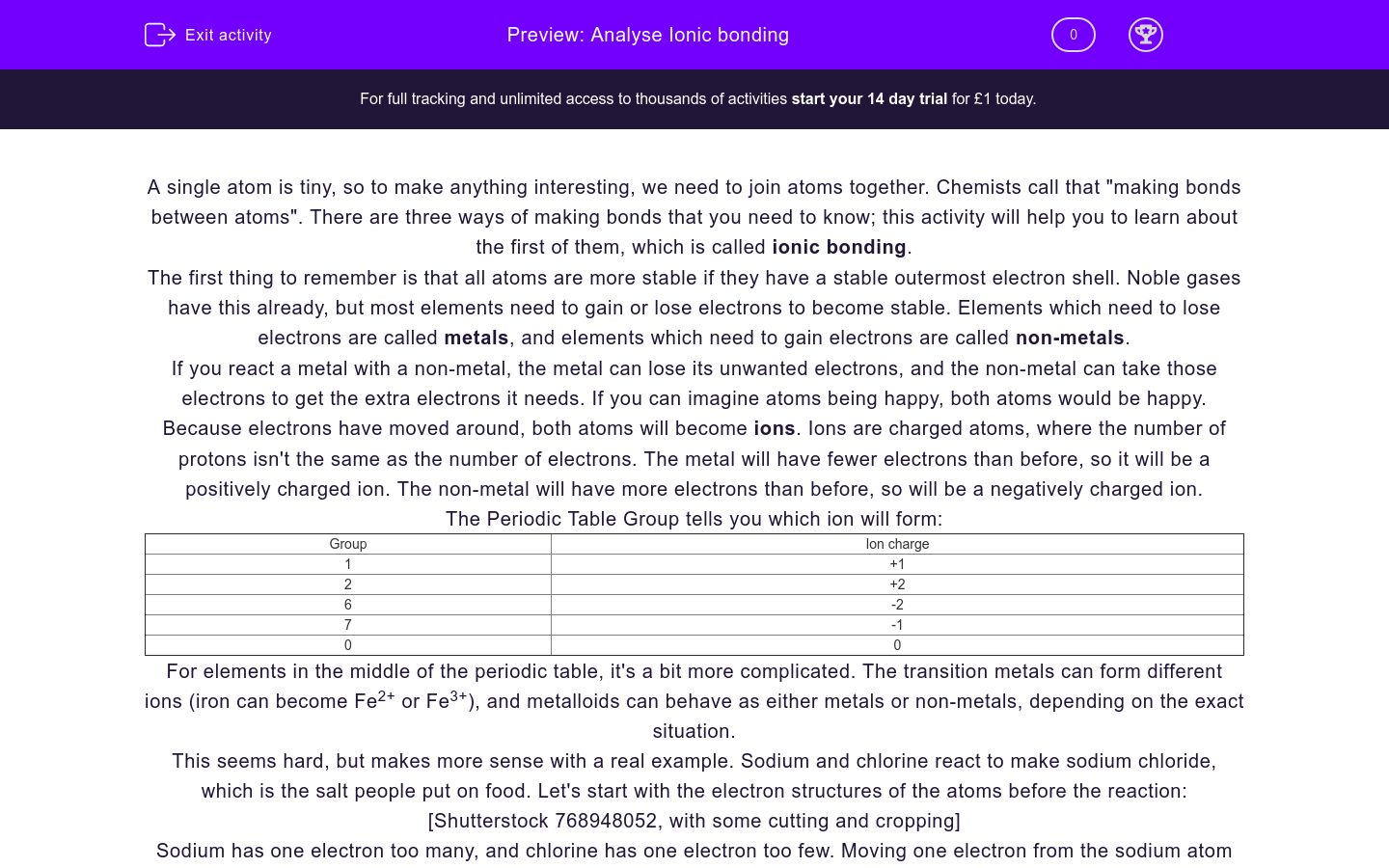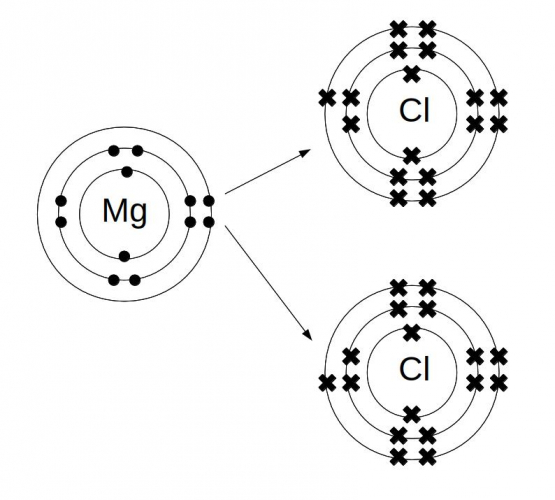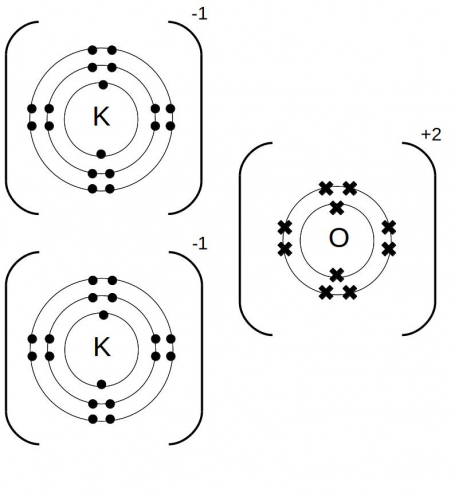# Analyse Ionic bonding

In this worksheet, students will learn how metals and non-metals react to make ionic compounds and how to predict formulas for ionic compounds.Key stage:  KS 4

GCSE Boards:   AQA, AQA Trilogy, OCR 21st Century, OCR Gateway, Pearson Edexcel, AQA Synergy

Difficulty level:### QUESTION 1 of 10

A single atom is tiny, so to make anything interesting, we need to join atoms together. Chemists call that "making bonds between atoms". There are three ways of making bonds that you need to know; this activity will help you to learn about the first of them, which is called ionic bonding.

The first thing to remember is that all atoms are more stable if they have a stable outermost electron shell. Noble gases have this already, but most elements need to gain or lose electrons to become stable. Elements which need to lose electrons are called metals, and elements which need to gain electrons are called non-metals.

If you react a metal with a non-metal, the metal can lose its unwanted electrons, and the non-metal can take those electrons to get the extra electrons it needs. If you can imagine atoms being happy, both atoms would be happy. Because electrons have moved around, both atoms will become ions. Ions are charged atoms, where the number of protons isn't the same as the number of electrons. The metal will have fewer electrons than before, so it will be a positively charged ion. The non-metal will have more electrons than before, so will be a negatively charged ion.

The Periodic Table Group tells you which ion will form:

 Group Ion charge 1 +1 2 +2 6 -2 7 -1 0 0

For elements in the middle of the periodic table, it's a bit more complicated. The transition metals can form different ions (iron can become Fe2+ or Fe3+), and metalloids can behave as either metals or non-metals, depending on the exact situation.

This seems hard, but makes more sense with a real example. Sodium and chlorine react to make sodium chloride, which is the salt people put on food. Let's start with the electron structures of the atoms before the reaction:

[Shutterstock 768948052, with some cutting and cropping]

Sodium has one electron too many, and chlorine has one electron too few. Moving one electron from the sodium atom to the chlorine atom makes both atoms into stable ions. The sodium has +1 charge (it's lost one negatively charged electron), and the chlorine has -1 charge (it's gained one negatively charged electron). The chlorine atom gets a new name; we call it a chloride ion.

You can write these processes as a pair of ionic equations:

Na - e- → Na+ (sodium atom loses an electron to make a positive sodium ion)

Cl + e- → Cl- (chlorine atom gains an electron to make a negative chloride ion)

Because the ions have opposite charges, they will be attracted to each other by electrostatic forces. That's why we talk about ionic bonding- the bond is an electrical force between different ions. Each positive ion attracts negative ions in all directions, so you get a repeating pattern which only stops when you run out of atoms. Ionic compounds have high melting and boiling points, because it needs a lot of energy to break the ionic bonds. If the ions can move, an ionic compound can conduct electricity- this happens in liquids (melts or solutions) but not solids.

We can show the way that electrons move to change atoms into ions using a dot and cross diagram. To see this, let's think about magnesium and chlorine reacting to make magnesium chloride.

Step 1: Draw the electron structure for the two elements you are reacting.

Magnesium has two electrons in its outermost shell, which it needs to lose. Chlorine has one empty slot in its outermost shell, which it needs to fill. In number form, the electron structure of Mg is 2.8.2 and Cl is 2.8.7.Step 2: Decide how many atoms you need of each element.

If each magnesium is losing two electrons, and each chlorine will only take one more, we need two chorines for each magnesium.(If we take another example, sodium nitride is Na3N. Nitrogen needs to gain 3 electrons, and sodium needs to lose 1 electron. That means we need electrons from three sodium atoms to complete the outer shell for one nitrogen atom.)

Step 3: Redraw the electron structures, showing the transferred electrons.

Don't try and edit your initial diagrams; you will end up with a confusing mess.Step 4: Put square brackets round the ions, and record their charges

The magnesium is Mg2+ (it's lost two electrons) and each chlorine is Cl- (each chlorine gains one electron).And that's it! Remember that ionic bonding happens between metals and non-metals, and works because the metal loses electrons, the non-metal gains electrons, and both atoms are more stable that way.

Fill in the gaps in this paragraph about electron shells. Use these words:

electrons

lose

negative

noble

non-metals

nothing

outermost

positive

shell

stable

Match these elements with the ions they form.

## Column B

Al
+ (or 1+)
Rb
- (or 1-)
Cl
2-
Se
3+

Which of these dot and cross diagrams shows lithium chloride?

 ABCDraw the dot and cross diagram for potassium oxide. Once you've tried drawing this for yourself, choose one of the options below.

 ABCWhich of these could be ionic compounds of oxygen? Tick all the correct possibilities.

LiO

BeO

Rb2O

Al2O3

CO2

Mg2O

Sort these part equations to make four complete ionic equations.

## Column B

Mg - 2e-
→Fe3+
Fe - 2e-
→O2-
Fe - 3e-
→Fe2+
O + 2e-
→Mg2+

What is the chemical formula of gallium sulfide? Type the right numbers in the boxes.

## Column B

Mg - 2e-
→Fe3+
Fe - 2e-
→O2-
Fe - 3e-
→Fe2+
O + 2e-
→Mg2+

What is the chemical formula of calcium nitride? Type the right numbers in the boxes.

## Column B

Mg - 2e-
→Fe3+
Fe - 2e-
→O2-
Fe - 3e-
→Fe2+
O + 2e-
→Mg2+

Which two ionic equations describe the formation of lithium phosphide?

Li - e- → Li+

Li - 2 e- → Li2+

P + 2 e- → PP + e- → P--

P + 2 e- → P2-

P + 3 e- → P3-

Mark this description of sodium oxide, by ticking all the correct sentences.

Sodium oxide is an ionic compound.

This is because oxygen is a metal, and sodium is a non-metal.

Sodium loses electrons to get a stable outer shell.

The ionic equation is Na - 2 e- → Na2+

Oxygen gains electrons, following the ionic equation O + 2 e- → O2-

To make the electron transfer balance, the formula of sodium oxide is Na2O.

The balanced equation for the reaction is 4 Na + O2 → 2 Na2O.

• Question 1

Fill in the gaps in this paragraph about electron shells. Use these words:

electrons

lose

negative

noble

non-metals

nothing

outermost

positive

shell

stable

EDDIE SAYS
Noble gases don't need to do anything, because they already have a stable outermost shell.
• Question 2

Match these elements with the ions they form.

## Column B

Al
3+
Rb
+ (or 1+)
Cl
- (or 1-)
Se
2-
EDDIE SAYS
You can work these out from their electron structures (Al is 2.8.3, so needs to lose 3 electrons, and so on), or by looking at the Groups in the Periodic Table.
• Question 3

Which of these dot and cross diagrams shows lithium chloride?

 ABCEDDIE SAYS
Lithium loses one electron, and oxygen gains two electrons. That means we need two lithiums for every oxygen. The electrons which move over keep their old symbol. The way I've drawn it, those are dots.
• Question 4

Draw the dot and cross diagram for potassium oxide. Once you've tried drawing this for yourself, choose one of the options below.

 ABCEDDIE SAYS
Potassium is in Group 1, so gives up 1 electron. Oxygen is in Group 6, so needs 2 electrons. That means that the formula is K2O.
• Question 5

Which of these could be ionic compounds of oxygen? Tick all the correct possibilities.

BeO
Rb2O
Al2O3
EDDIE SAYS
LiO doesn't work, because each oxygen needs two electrons, and each lithium only gives up one- you need Li2O instead. Carbon dioxide is a valid compound, but it isn't ionic; carbon and oxygen are both non-metals. Magnesium oxide is MgO, not Mg2O.
• Question 6

Sort these part equations to make four complete ionic equations.

## Column B

Mg - 2e-
→Mg2+
Fe - 2e-
→Fe2+
Fe - 3e-
→Fe3+
O + 2e-
→O2-
EDDIE SAYS
The tricky bit is remembering that adding electrons makes a negative ion, and removing electrons makes a positive ion. Once you\'ve got the hang of that, you just need to make sure that you keep track of the number of electrons.
• Question 7

What is the chemical formula of gallium sulfide? Type the right numbers in the boxes.

EDDIE SAYS
Ga is in group 3, so it needs to lose 3 electrons. Sulfur is in group 6, so it needs to gain 2 electrons. To make the electron transfer work, you need 2 gallium atoms giving electrons to 3 sulfur atoms. In total, 6 electrons get transferred.
• Question 8

What is the chemical formula of calcium nitride? Type the right numbers in the boxes.

EDDIE SAYS
Ca is in group 2, so it needs to lose 2 electrons. Nitrogen is in group 5, so it needs to gain 3 electrons. To make the electron transfer work, you need 3 calcium atoms giving electrons to 2 nitrogen atoms. In total, 6 electrons get transferred.
• Question 9

Which two ionic equations describe the formation of lithium phosphide?

Li - e- → Li+
P + 3 e- → P3-
EDDIE SAYS
The numbers of electrons in the ionic equations depend on the ions you are making. Balancing the pair of equations is the next step; since each Li atom only gives up one electron, you need three lithium ions formed for to form one phosphide ion.
• Question 10

Mark this description of sodium oxide, by ticking all the correct sentences.

Sodium oxide is an ionic compound.
Sodium loses electrons to get a stable outer shell.
Oxygen gains electrons, following the ionic equation O + 2 e- → O2-
To make the electron transfer balance, the formula of sodium oxide is Na2O.
The balanced equation for the reaction is 4 Na + O2 → 2 Na2O.
EDDIE SAYS
Remember that metals lose electrons, and non-metals gain them, to form ions with a stable outer shell. To get the right ionic equations, think about how many electrons an atom has to gain or lose. The last statement is tricky, because oxygen gas has molecules with two atoms. You'll find out why when you learn about covalent bonding.
---- OR ----

Sign up for a £1 trial so you can track and measure your child's progress on this activity.

### What is EdPlace?

We're your National Curriculum aligned online education content provider helping each child succeed in English, maths and science from year 1 to GCSE. With an EdPlace account you’ll be able to track and measure progress, helping each child achieve their best. We build confidence and attainment by personalising each child’s learning at a level that suits them.

Get started